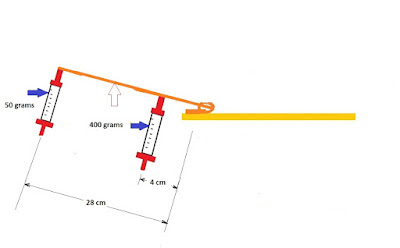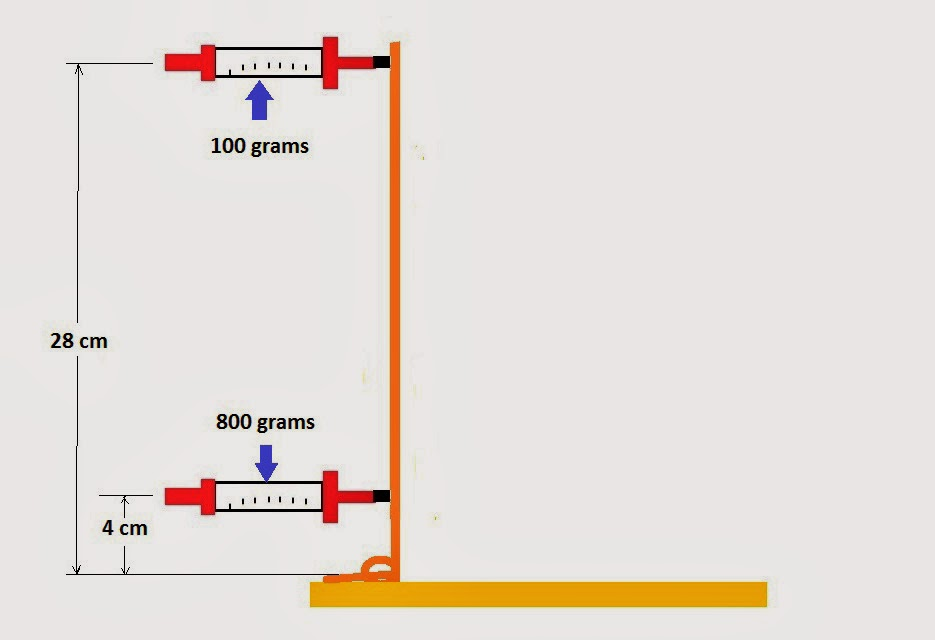## Thursday, March 20, 2014

### Torque and Lever Arm Calculations for a Mousetrap Car

Torque has been defined either as a twisting force or the tendency to rotate around an axis.  A common example of torque is tightening a bolt with a wrench.  To know how much torque is being applied to a bolt a mechanics will often use a special type of wrench known as a “torque wrench” so a specified amount of torque can be applied to a bolt.

The formula for torque is very simple if the force is applied perpendicular to the lever: Torque = radius x force.  Normally units of torque are foot pounds or newton meters.  This equation gives the torque applied to the pivot point, this is the mechanical advantage concept.

I have been thinking more about how math could be used to predict an outcome. To start with a mousetrap car if you could calculate what force is available at the end of the lever arm based on the torque at the axis it would give you some idea on how much force is available to propel the mousetrap car.  It is also interesting to see how the force decreases as the spring unwinds.

For a mousetrap car that will go a long distance the lever arm needs to be longer to pull more line that is wrapped around the driving axle.  From these calculations it can be easily seen that the amount of force available rapidly diminishes as the length of the lever arm increases.

To me it is also interesting if you can measure calculated outcomes and think about the reasons for inaccuracies.  For this experiment inaccuracy was related to the spring scale I was using and how I was using it.  Doc Fizzix sells a torsion wheel to measure the torque of the mousetrap spring.  Link to Torsion Wheel product.

For my experiment I measured the force in grams at 4 centimeters from the axis at 25, 90, and 180 degrees. I then measured at 28 centimeters from the axis and then calculated what the force should be based on the measurements at 4 centimeters.

Above are the comparisons of the measurements and the calculations which appear in red.Measured Forces on Lever Arm at 25 DegreesMeasured Forces on Lever Arm at 90 DegreesMeasured Forces on Lever Arm at 180 Degrees

Bill Kuhl

New Mousetrap Car Article on Website

For an explanation of many aspects of simple aerodynamics with math problems check out my article Basic Aerodynamics With a Lesson

#### 1 comment:

1.Even it is just a mousetrap car but still it follows the same principle that a real car does. In order to move it perfectly, there should be a proper balance between the torque and the level arm. Thanks for elaborating the concept. For detail logic of a vehicle it is better to talk to a mechanic. Mini Cooper Specialist Beaverton, OR. They can make the doubts clear by providing you real-life examples. Anyways, keep posting.